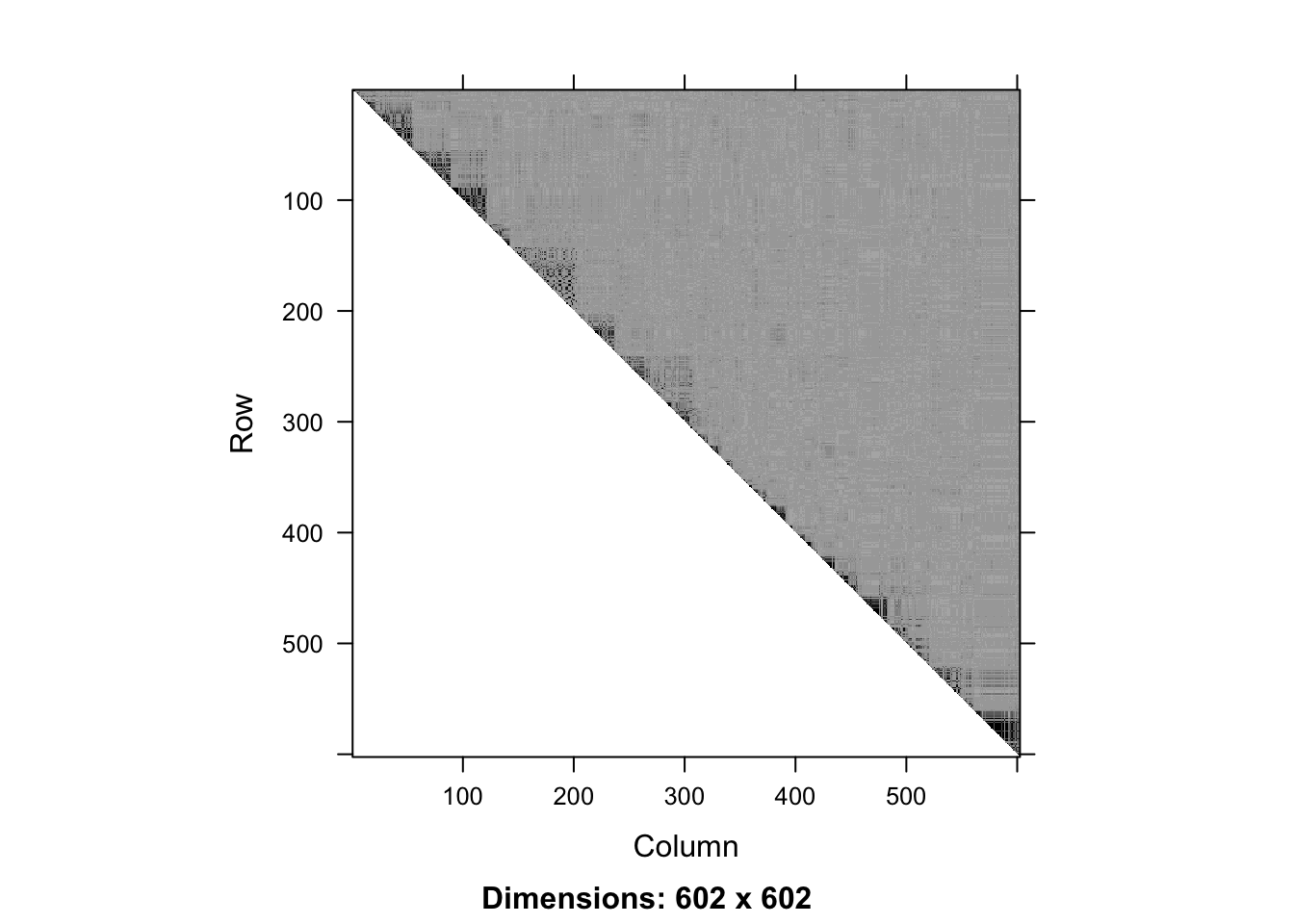``````# this vignette is not created if snpStats is not installed
if (!require("snpStats")) {
knitr::opts_chunk\$set(eval = FALSE)
}``````
``## Loading required package: snpStats``
``## Loading required package: survival``
``## Loading required package: Matrix``
``````##
## Attaching package: 'Matrix'``````
``````## The following object is masked from 'package:S4Vectors':
##
##     expand``````

# Introduction

In this vignette we demonstrate the use of `snpClust` function in the `adjclust` package. `snpClust` performs adjacency-constrained hierarchical clustering of single nucleotide polymorphisms (SNPs), where the similarity between SNPs is defined by linkage disequilibrium (LD).

This function implements the algorithm described in the third chapter of . It is an extension of the algorithm described in . Denoting by \(p\) the number of SNPs to cluster and assuming that the similarity between SNPs whose indices are more distant than \(h\), its time complexity is \(O(p (\log(p) + h))\), and its space complexity is \(O(hp)\).

``library("adjclust")``

The beginning of this vignette closely follows the "LD vignette" of the SnpStats package . First, we load genotype data.

``data("ld.example", package = "snpStats")``

We focus on the `ceph.1mb` data.

``````geno <- ceph.1mb[, -316]  ## drop one SNP leading to one missing LD value
p <- ncol(geno)
nSamples <- nrow(geno)
geno``````
``````## A SnpMatrix with  90 rows and  602 columns
## Row names:  NA06985 ... NA12892
## Col names:  rs5993821 ... rs5747302``````

These data are drawn from the International HapMap Project and concern 602 SNPs1 over a 1Mb region of chromosome 22 in sample of 90 Europeans.

We can compute and display the LD between these SNPs.

``````ld.ceph <- snpStats::ld(geno, stats = "R.squared", depth = p-1)
image(ld.ceph, lwd = 0)``````The `snpClust` function can handle genotype data as an input:

``fit <- snpClust(geno, stats = "R.squared")``
``````## Warning in run.snpClust(x, h = h, stats = stats): Forcing the LD similarity to
## be smaller than or equal to 1``````
``## Note: 135 merges with non increasing heights.``

Note that due to numerical errors in the LD estimation, some of the estimated LD values may be slightly larger than 1. These values are rounded to 1 internally.

The above figure suggests that the LD signal is concentrated close to the diagonal. We can focus on a diagonal band with the bandwidth parameter `h`:

``fitH <- snpClust(geno, h = 100, stats = "R.squared")``
``````## Warning in run.snpClust(x, h = h, stats = stats): Forcing the LD similarity to
## be smaller than or equal to 1``````
``## Note: 133 merges with non increasing heights.``
``fitH``
``````##
## Call:
## run.adjclust(mat = mat, type = type, h = h, strictCheck = strictCheck)
##
## Cluster method   : snpClust
## Number of objects: 602``````

# Output

The output of the `snpClust` is of class `chac`. In particular, it can be plotted as a dendrogram silently relying on the function `plot.dendrogram`:

``plot(fitH, type = "rectangle", leaflab = "perpendicular")``
``````## Warning in plot.chac(fitH, type = "rectangle", leaflab = "perpendicular"):
## Detected reversals in dendrogram: mode = 'corrected', 'within-disp' or 'total-disp' might be more relevant.``````